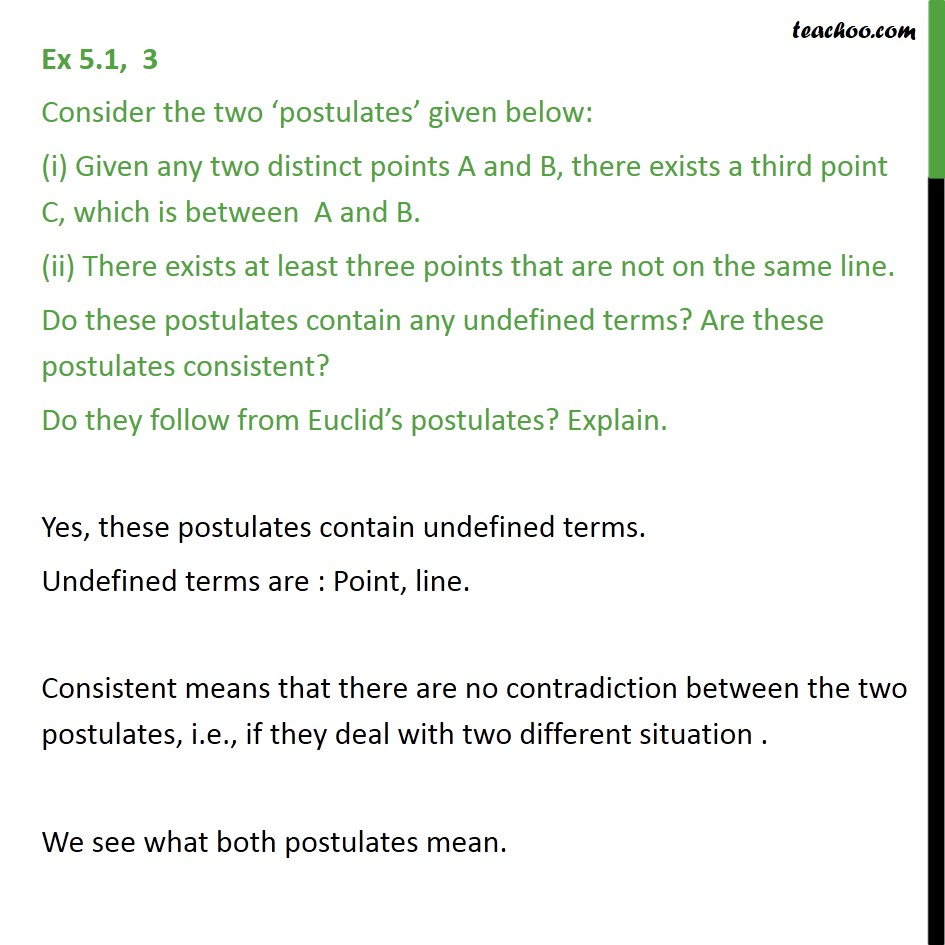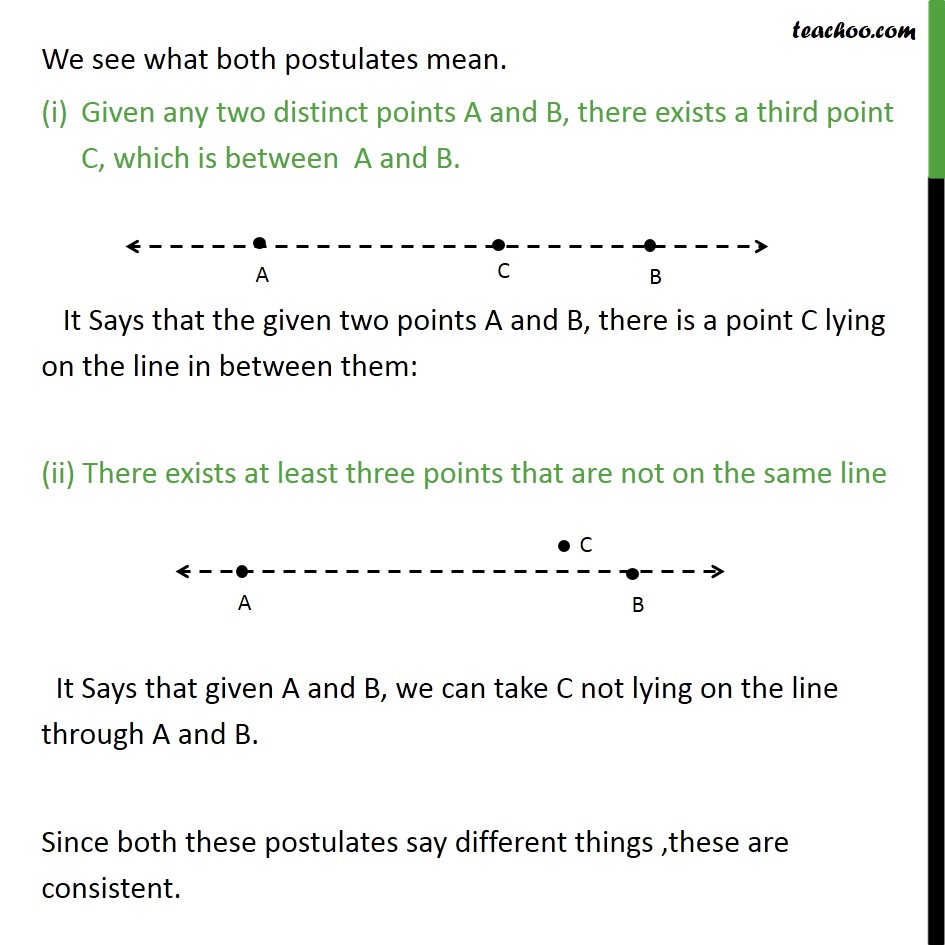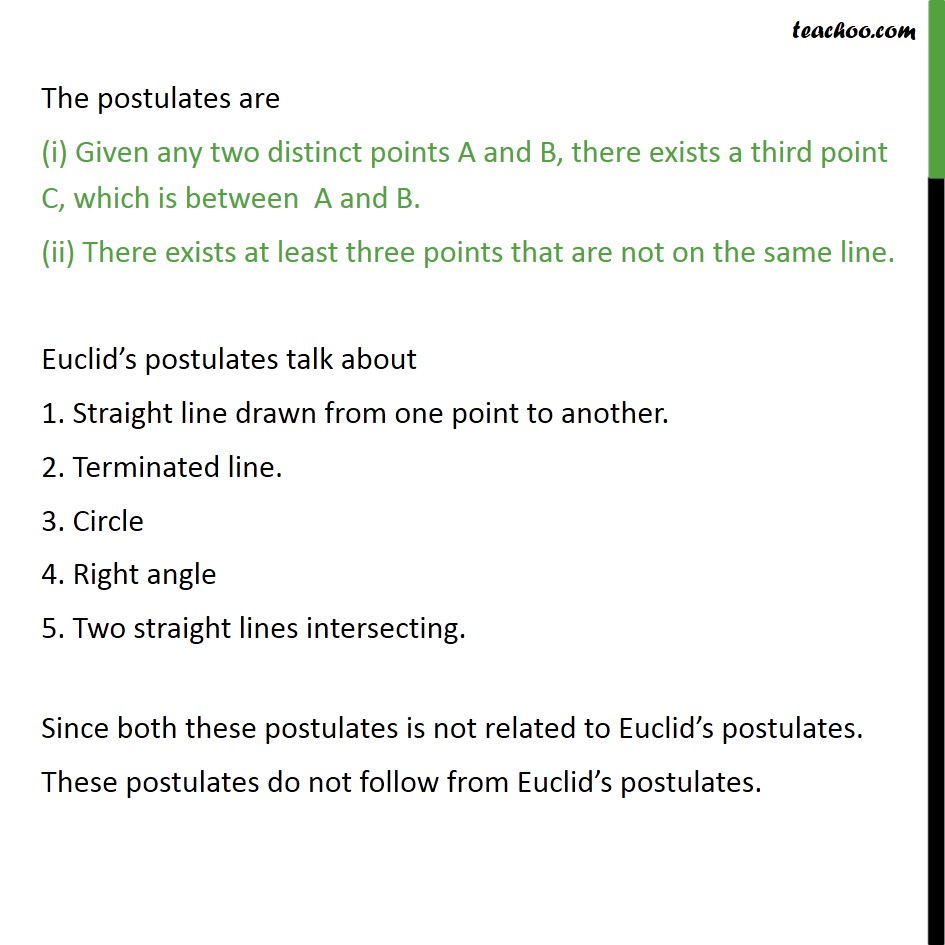Ex 5.1

Chapter 5 Class 9 Introduction to Euclid's Geometry
Serial order wiseMaths Crash Course - Live lectures + all videos + Real time Doubt solving!

### Transcript

Ex 5.1, 3 Consider the two postulates given below: (i) Given any two distinct points A and B, there exists a third point C, which is between A and B. (ii) There exists at least three points that are not on the same line. Do these postulates contain any undefined terms? Are these postulates consistent? Do they follow from Euclid s postulates? Explain. Yes, these postulates contain undefined terms. Undefined terms are : Point, line. Consistent means that there are no contradiction between the two postulates, i.e., if they deal with two different situation . We see what both postulates mean. We see what both postulates mean. Given any two distinct points A and B, there exists a third point C, which is between A and B. It Says that the given two points A and B, there is a point C lying on the line in between them: (ii) There exists at least three points that are not on the same line It Says that given A and B, we can take C not lying on the line through A and B. Since both these postulates say different things ,these are consistent. The postulates are (i) Given any two distinct points A and B, there exists a third point C, which is between A and B. (ii) There exists at least three points that are not on the same line. Euclid s postulates talk about 1. Straight line drawn from one point to another. 2. Terminated line. 3. Circle 4. Right angle 5. Two straight lines intersecting. Since both these postulates is not related to Euclid s postulates. These postulates do not follow from Euclid s postulates.